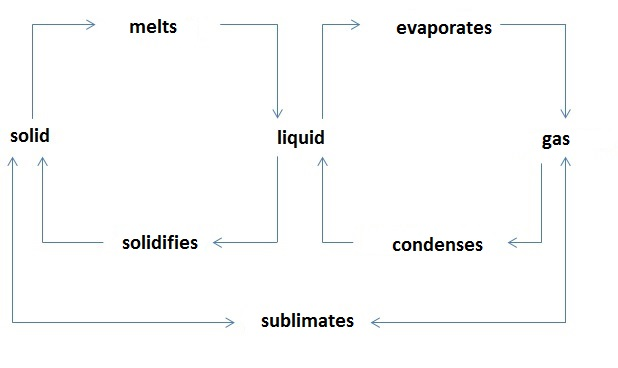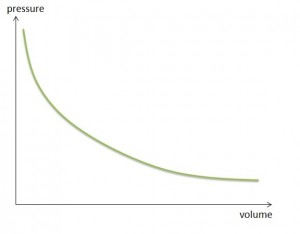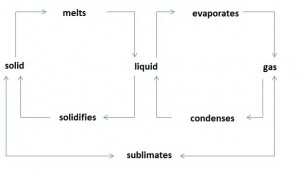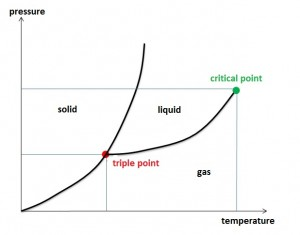Are you more of a visual learner? Check out our online video lectures and start your physics course now for free!Thermodynamic Variables—Pressure, Volume, and Temperature

Pressure Propagation in Liquids and Gases

 p pressure [Pa], [N/m2] F force acting on a surface[N] A area of the surface, cross-section [m2]

Liquids have no definite shape as the molecules and atoms move around freely, and they assume the shape of the available vessel. As a whole, the liquid surface forms a horizontal plane. Gases lack binding forces between the individual molecules, which are constantly in motion, except at the temperature of absolute zero. They are repelled by collision or bounce against the surrounding walls. Thus, they move in irregular zigzag paths.

A gas molecule undergoes nearly 10 quadrillion collisions per second.

In a fully sealed vessel, force is exerted on a fluid. Unlike solids, where a force is exerted at a single point, the particles in a fluid avoid contact due to their mobility. When the force is evenly distributed through the entire surface, the particles adjacent to the surface experience immediate pressure, which is transmitted in all directions due to their mobility. Therefore, the pressure is the same at any point within a fluid.

The pressure is defined as the ratio of the force applied perpendicular to a surface and the area of this surface: P = F / A.

Pressure and Volume of a Gas

The volume and pressure of a gas are inversely correlated. At constant temperature, the pressure of a gas is proportional to the number of molecules present in the respective area. The mass of gas is a measure of the number of molecules.

Boyle-Mariotte law:
At constant temperature and in a closed container, the product of pressure and volume of a given amount of gas is constant.
• PV=constant
• P1V1 = P2V2Image: Volume pressure Boyle Mariotte. By Lecturio

Since a change in pressure affects the gas volume, the density of gas depends on the pressure as well. At constant temperature, the density of a gas is proportional to the pressure. Densities behave similar to pressures.

Real and Ideal Gases

Real gases have a volume and thus occupy space. They collide with each other and their motion is slowed or increased. Their particles are in constant interaction with each other.

The particles in a gas exhibit the following features:

• Particles are elastic bodies of negligible small volumes.
• Elastic reflections occur at the vessel walls.
• Particles are evenly distributed (homogeneity).
• The particle velocities are evenly distributed (isotropy).

Ideal gases have no definite volume or mass, and their particle collisions are elastic. During particle collision, no energy is involved and the particle pressure is higher compared to that of ideal gases.

Equation of State for Ideal Gases

 V Volume [l] m Mass [kg] M Molar mass n Amount of substance [mol] R Universal gas constant [J/(K*mol)] T Temperature [K], [°C]

For a description of ideal gases, a general equation of state (the ideal gas law or the general gas equation) is used. It is defined as the simultaneous change of volume, pressure, and temperature.

p * V = m * R * T

In this equation, the absolute pressure is used. The ideal gas law does not apply under conditions of excessive pressure. Further, the general gas equation is only applicable to ideal gases, with limited validity for real gases and none whatsoever for vapors. Based on the ideal gas law, the standard volume of material can be calculated. Standard volume refers to the volume at standard atmospheric pressure (p0) defined as 1013 mbar and at a temperature (T) of 0°C. Under these standard conditions (STP), 1 mole of an ideal gas occupies a volume of V= 22.4 L.

If the constant p * V / T is proportional to the mass of the closed system, the associated proportionality factor is referred to as gas constant. The value of the gas constant depends on the type of gas.

The number of similar particles contained in a system is described as the amount of substance. A mole is the amount of a substance containing as many particles as there are atoms in 12 grams of a carbon isotope C12. Therefore, 1 mole of any substance contains 6.0221367 * 1023 particles. Under standard conditions, 1 mole of any gas contains the same volume (see standard volume).

The product of universal gas constant with molar mass is known as the molar gas constant. It is a universal constant and thus non-specific.

p * V = n * M * R * T

The equation of state in relation to the amount of substance is expressed as:

p * V = n * R * T

Thus, the state of a gas is determined by 3 variables: pressure, volume, and temperature. Changes in 2 or all state variables are referred to as changes of state.

The internal energy for each state of a system is clearly defined. These changes are defined by the First Law of Thermodynamics (see the article Thermodynamics I):

The supply of heat and mechanical work increases the internal energy of a closed system based on the equation:

ΔU = Q + W

In ideal gases, mechanical work triggers a volume change. The thermal energy supplied leads to increased internal energy and volume.

 Isothermal change of state Change of state of an ideal gas is isothermal at a constant temperature. p * V = constant Isobaric change of state Change of state of an ideal gas is isobaric at constant pressure. V / T = constant Isochoric change of state Change of state of an ideal gas is isochoric at constant volume. ρ / T = constant

 K Adiabatic exponent ΔS Change of entropy (S = 0 in adiabatic change) Cp Isobaric heat capacity [J/(kg*K)] CV Isochoric heat capacity [J/(kg*K)]

Adiabatic change of an ideal gas occurs without heat exchange with the environment. Complete heat insulation is required to generate this altered state. Adiabatic changes of state are also referred to as isentropic changes of state defined by the corresponding equation:

p * VK = const; K = CP / CV

The work performed in an isentropic relaxation for a specific amount of gas only depends on the change in temperature.

Gas Mixtures

 ρA Partial pressure of a gas [bar] A Concentration of a gas in a solvent [mole/l] K Constant

Mixtures of at least 2 different chemical elements that result in a gas are called gas mixtures. They show a homogeneous mixing ratio.

The solubility of a gas in a liquid is defined by Henry’s law. The gas concentration in the liquid is proportional to the partial pressure of the gas above the liquid.

A = K · pA

States of Aggregation

Materials can assume different states of aggregation:

• Solid
• Liquid
• GasImage: Overview of physical state change. By Lecturio

Each of these 3 states of matter is associated with specific properties. Solids have a fixed shape and volume. Their particles are held together by cohesive forces. Liquids have no fixed shape and take the shape of their container. However, they have a fixed volume, with cohesive forces acting between the particles. Gaseous substances neither possess a definite shape nor a specific volume. Their particles exert only insignificant cohesive forces. These are the primary states of matter as they all occupy space and carry weight despite the differences in terms of cohesive bonds between the particles.

Energy is required to reach a higher state of aggregation (solid < liquid < gaseous), whereas energy is released when a lower state of aggregation or disaggregation occurs.

Melting and Solidification

The transition from a solid into a liquid state is called melting, whereas the reverse change is termed solidification.

Melting of a body occurs at its melting temperature. Melting and solidification temperatures are the same for a substance. During melting, the temperature remains constant despite the addition of heat to transform the material from solid to liquid state. Similarly, the solidification temperature remains constant despite the release of heat. Only after the solidification of the entire substance, further heat dissipation results in cooling of the solid. These 2 processes explain the disruptive effects of heat energy on the cohesive bonds in solids, resulting in conversion to a liquid state.

Vaporization and Condensation

During vaporization, a substance changes from a liquid to a gaseous state. Condensation entails the reverse process.

There are 2 types of vaporization: Boiling involves the evaporation of a liquid when vapor bubbles form inside the liquid phase. The temperature of the liquid remains constant during boiling despite further heat supply. The boiling temperature is strongly dependent on the pressure of the liquid. The normal boiling temperature refers to the boiling temperature at atmospheric pressure of 1013 mbar. In the other type of vaporization known as evaporation, a liquid vaporizes below its boiling temperature. Evaporation occurs only on the liquid surface and at every temperature above 0. During evaporation, a liquid derives the required heat from its intrinsic energy and from the environment.

Example: When a person is sweating, the sweat evaporates from the surface of the skin. The energy in the form of heat is released. The body releases excess heat to prevent overheating.

‘Pure’ water is generated via evaporation and condensation of water during liquid treatment known as distillation. Distillation is generally recommended to purify water for drinking at home and for commercial applications.

Sublimation

Many substances change directly from the solid to the gaseous state and vice versa, without any form of liquid, for e.g., the volatilization of naphthalene or the formation of ice crystals in the air. This phase transitions directly from solid to gas and vice versa is called sublimation.

Vapor Pressure

In the case of ideal gases, pressure and volume are inversely proportional to each other. In the case of real gases, this inverse relationship is only accurate by approximation. When vapors change in volume, the pressure hardly changes. This difference between gases and vapors is based on the variation in temperature and pressure-dependent boiling point.

Vapor pressure (p) does not depend on the volume of the vapor. It only depends on the temperature.

The vapor is saturated when the saturated vapor pressure is reached, i.e., when it is at equilibrium with the liquid pressure.

Gases are highly unsaturated vapors, with temperature is far above the boiling point that corresponds to their pressure.

Water Vapor in the Air

Since water constantly evaporates from the surface of all-natural bodies of water, the air always contains water vapor, which is mostly unsaturated. To become saturated, it must be cooled to a specific temperature known as the dew point. In case of cooling below the dew point, large water vapor is condensed so that only the water vapor corresponding to this temperature remains. The condensed water vapor appears in the form of fog, rain, snow or frost.

At each temperature, only a certain amount of water vapor can exist in a certain volume of air. The water vapor in the atmospheric air varies with time and location and is referred to as humidity. The maximum humidity is defined as the maximum amount of water vapor contained in one cubic meter of air at a certain temperature.

F (maximum) = Mass of water vapor in the air / Volume of humid air

Critical Temperature

The critical temperature of a gas is the temperature at which liquefaction is no longer possible, regardless of the increased pressure level. The corresponding isothermal curve shows an inflection point with a horizontal tangent at the critical pressure. Only below the critical temperature gases can be liquefied under pressure.

Real gases slightly cool down during reduced relaxation.

Triple PointImage by Lecturio

The three states of aggregation can reach thermodynamic equilibrium. When this equilibrium, defined by pressure and temperature, exists, it is known as triple point.

Learn. Apply. Retain.
Your path to achieve medical excellence.
Study for medical school and boards with Lecturio.